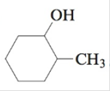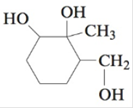Chapter 22, Problem 65E

Chapter
Section
Textbook Problem

# Using appropriate reactants, alcohols can be oxidized into aldehydes, ketones, and/or carboxylic acids. Primary alcohols can be oxidized into aldehydes, which can then be oxidized into carboxylic acids. Secondary alcohols can be oxidized into ketones, while tertiary alcohols do not undergo this type of oxidation. Give the structure of the product(s) resulting from the oxidation of each of the following alcohols.a. 3-metbyl-1-butanolb. 3-methyl-2-butanolc. 2-methyl-2-butanold.e.f.(a)

Interpretation Introduction

Interpretation: The structures for the products of the given oxidation reactions of alcohols are to be drawn.

Concept introduction: Alcohols are oxidized to either aldehyde or carboxylic acids depending upon the reaction condition. When the formation of carboxylic acids is required, primary alcohol is first oxidizing to aldehyde which is then further oxidizes to give carboxylic acids. Secondary alcohols can be oxidized into ketones and tertiary alcohols do not undergo this type of oxidation.

To determine: The structure for the product of the given oxidation reaction of alcohol.

Explanation

Explanation

The product is 3-methylbutanoicacid whose structure is shown in Figure 1.

The given reactant is 3-ethyl-1-butanol a primary alcohol and on oxidation first it conv

(b)

Interpretation Introduction

Interpretation: The structures for the products of the given oxidation reactions of alcohols are to be drawn.

Concept introduction: Alcohols are oxidized to either aldehyde or carboxylic acids depending upon the reaction condition. When the formation of carboxylic acids is required, primary alcohol is first oxidizing to aldehyde which is then further oxidizes to give carboxylic acids. Secondary alcohols can be oxidized into ketones and tertiary alcohols do not undergo this type of oxidation.

To determine: The structure for the product of the given oxidation reaction of alcohol.

(c)

Interpretation Introduction

Interpretation: The structures for the products of the given oxidation reactions of alcohols are to be drawn.

Concept introduction: Alcohols are oxidized to either aldehyde or carboxylic acids depending upon the reaction condition. When the formation of carboxylic acids is required, primary alcohol is first oxidizing to aldehyde which is then further oxidizes to give carboxylic acids. Secondary alcohols can be oxidized into ketones and tertiary alcohols do not undergo this type of oxidation.

To determine: The structure for the product of the given oxidation reaction of alcohol.

(d)

Interpretation Introduction

Interpretation: The structures for the products of the given oxidation reactions of alcohols are to be drawn.

Concept introduction: Alcohols are oxidized to either aldehyde or carboxylic acids depending upon the reaction condition. When the formation of carboxylic acids is required, primary alcohol is first oxidizing to aldehyde which is then further oxidizes to give carboxylic acids. Secondary alcohols can be oxidized into ketones and tertiary alcohols do not undergo this type of oxidation.

To determine: The structure for the product of the given oxidation reaction of alcohol.

(e)

Interpretation Introduction

Interpretation: The structures for the products of the given oxidation reactions of alcohols are to be drawn.

Concept introduction: Alcohols are oxidized to either aldehyde or carboxylic acids depending upon the reaction condition. When the formation of carboxylic acids is required, primary alcohol is first oxidizing to aldehyde which is then further oxidizes to give carboxylic acids. Secondary alcohols can be oxidized into ketones and tertiary alcohols do not undergo this type of oxidation.

To determine: The structure for the product of the given oxidation reaction of alcohol.

(f)

Interpretation Introduction

Interpretation: The structures for the products of the given oxidation reactions of alcohols are to be drawn.

Concept introduction: Alcohols are oxidized to either aldehyde or carboxylic acids depending upon the reaction condition. When the formation of carboxylic acids is required, primary alcohol is first oxidizing to aldehyde which is then further oxidizes to give carboxylic acids. Secondary alcohols can be oxidized into ketones and tertiary alcohols do not undergo this type of oxidation.

To determine: The structure for the product of the given oxidation reaction of alcohol.

### Still sussing out bartleby?

Check out a sample textbook solution.

See a sample solution

#### The Solution to Your Study Problems

Bartleby provides explanations to thousands of textbook problems written by our experts, many with advanced degrees!

Get Started